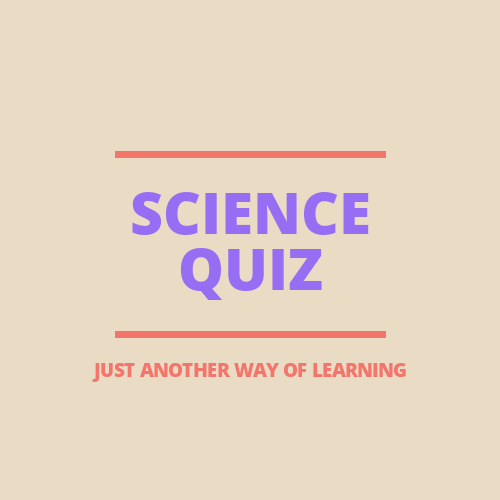9
Created on ByScience & TechnologySCIENCE

science quiz contain 15 multiple choice question with one correct answer,

1 / 15

What is used for measure very small intervals are precisely

2 / 15

A chronometer measures?

3 / 15

One Joule is equal to____ eargs

4 / 15

What is used as moderator in nuclear reactor?

5 / 15

Nautical mile is unit of distance used in which of the following

6 / 15

Bar is the unit of which?

7 / 15

What is used for measurement of electric current

8 / 15

KHz(Kilohertz) is a unit for measure?

9 / 15

How many miles are there in one kilometer?

10 / 15

Atoms are composed of ?

11 / 15

Power is measure by which unit?

12 / 15

Speed of ______ measure by Knot:

13 / 15

Which one is positively charge particle?

14 / 15

One Horse Power( 1 HP) is equal to ?

15 / 15

Light year is a measurement of which